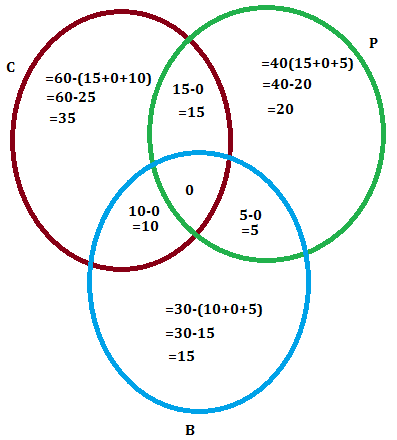QUESTION

# In a college, 60 students enrolled in chemistry, 40 in physics, 30 in biology, 15 in chemistry and physics, 10 in physics and biology, 5 in biology and chemistry. No one enrolled in all the three. Find how many are enrolled in at least one of the subjects.

Hint: In this question, find the number of students enrolled in at least one of the subjects first work out the sum of students with all three subjects, with two subjects only and with only one subject. Second, work out the no of students enrolled in a single subject only and finally work out the different values of each subject.
Complete step-by-step solution -
Let C, P and B are the students enrolled in the subjects Chemistry, Physics and Biology respectively.
Number of students enrolled in the subject Chemistry = $n(C)=60$
Number of students enrolled in the subject Physics = $n(P)=40$
Number of students enrolled in the subject Biology = $n(B)=30$

Number of students enrolled in the subjects chemistry and Physics = $n(C\bigcap P)=15$

Number of students enrolled in the subjects Physics and Biology = $n(P\bigcap B)=10$

Number of students enrolled in the subjects Biology and chemistry = $n(B\bigcap C)=5$

Number of students enrolled in the subjects Physics Biology and Chemistry = $n(P\bigcap B\bigcap C)=0$Therefore, the number of students enrolled in at least one of the subjects = 35 + 15 + 20 + 10 + 5 + 15 = 100.

Note: Alternatively, you could substitute all the number of the students in the subjects Chemistry, Physics and Biology directly into the formula $n\left( C\bigcup P\bigcup B \right)=n(C)+n(P)+n(B)-n(C\bigcap P)-n(P\bigcap B)-n(C\bigcap B)+n(C\bigcap P\bigcap B)$